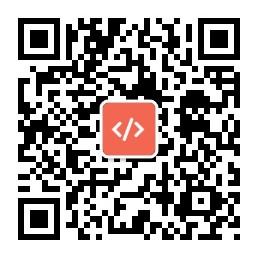# 节点使用教程1、弹幕效果

<svg width="100%" height="25" xmlns="http://www.w3.org/2000/svg">

<g>

<text font-family="microsoft yahei,sans-serif" font-size="20" y="20" x="100%" fill="#898989">

IT之家

<animate attributename="x" from="100%" to="-200" begin="0s" dur="3s" repeatcount="indefinite"></animate>

</text>

</g>

</svg>

2、改变透明度

<center>

<svg xmlns="http://www.w3.org/2000/svg" version="1.1">

<text x="35%" y="20" fill="#898989" font-family="microsoft yahei,sans-serif" font-weight="bold" font-size="14">

IT之家            <animate attributename="opacity" from="1" to="0" begin="0s" dur="1.2s" repeatcount="indefinite"></animate>

</text>

<text x="35%" y="40" fill="#767676" font-family="microsoft yahei,sans-serif" font-weight="bold" font-size="14">

IT之家            <animate attributename="opacity" from="1" to="0" begin="0.2s" dur="1.2s" repeatcount="indefinite"></animate>

</text>

<text x="35%" y="60" fill="#646464" font-family="microsoft yahei,sans-serif" font-weight="bold" font-size="14">

IT之家            <animate attributename="opacity" from="1" to="0" begin="0.4s" dur="1.2s" repeatcount="indefinite"></animate>

</text>

<text x="35%" y="80" fill="#333333" font-family="microsoft yahei,sans-serif" font-weight="bold" font-size="14">

IT之家            <animate attributename="opacity" from="1" to="0" begin="0.6s" dur="1.2s" repeatcount="indefinite"></animate>

</text>

<text x="35%" y="100" fill="#000000" font-family="microsoft yahei,sans-serif" font-weight="bold" font-size="14">

IT之家            <animate attributename="opacity" from="1" to="0" begin="0.8s" dur="1.2s" repeatcount="indefinite"></animate>

</text>

</svg>

</center>

3、结合GIF实现图片弹幕效果：

①  首先，我们可以选取一张gif图片上传到微博②  点击查看大图 ― 在新标签页中打开图片，获得该图片的网页链接③  将如下代码复制到微信HTML编辑框中，代码中的图片链接可使用刚才获得的图片链接，汉字部分用户可自行替换成其他：

<p>

<img src="http://ww2.sinaimg.cn/mw1024/b96366fbjw1eyyee3vv2tg20dw07t7h7.gif"/>

</p>

<svg width="100%" height="200" xmlns="http://www.w3.org/2000/svg" style="margin-top: -160px;">

<text font-family="microsoft yahei,sans-serif" font-size="18" y="20" x="5%" fill="#c00000" font-weight="bold">

IT之家

<animate attributename="opacity" from="1" to="0" begin="0.8s" dur="5s" repeatcount="indefinite"></animate>

</text>

<text font-family="microsoft yahei,sans-serif" font-size="14" y="20" x="109.055" fill="#ffffff" font-weight="bold">

IT之家

<animate attributename="x" from="800" to="-400" begin="0s" dur="5.5s" repeatcount="indefinite"></animate>

</text>

<text font-family="microsoft yahei,sans-serif" font-size="14" y="34" x="109.055" fill="#ffffff" font-weight="bold">

IT之家

<animate attributename="x" from="800" to="-400" begin="0s" dur="5.5s" repeatcount="indefinite"></animate>

</text>

<text font-family="microsoft yahei,sans-serif" font-size="14" y="49" x="109.055" fill="#ffffff" font-weight="bold">

IT之家

<animate attributename="x" from="800" to="-400" begin="0s" dur="5.5s" repeatcount="indefinite"></animate>

</text>

<text font-family="microsoft yahei,sans-serif" font-size="14" y="28" x="283.601" fill="#ffffff" font-weight="bold">

IT之家

<animate attributename="x" from="800" to="-400" begin="0.8s" dur="5.5s" repeatcount="indefinite"></animate>

</text>

<text font-family="microsoft yahei,sans-serif" font-size="14" y="110" x="24%" fill="#ffffff" font-weight="bold">

IT之家

<animate attributename="opacity" from="1" to="0" begin="1.6s" dur="1s" repeatcount="indefinite"></animate>

</text>

<text font-family="microsoft yahei,sans-serif" font-size="14" y="130" x="24%" fill="#ffffff" font-weight="bold">

IT之家

<animate attributename="opacity" from="1" to="0" begin="1.2s" dur="1s" repeatcount="indefinite"></animate>

</text>

<text font-family="microsoft yahei,sans-serif" font-size="14" y="150" x="24%" fill="#ffffff" font-weight="bold">

IT之家

<animate attributename="opacity" from="1" to="0" begin="0.8s" dur="1s" repeatcount="indefinite"></animate>

</text>

</svg>1、点击全文查看的段子

1、首先在朋友圈发消息界面将你想要发送的段子打好，未必一定是两句话，但最好精辟，这样才有效果。将要隐藏在点击全文后才能看到的话单独一行显示。2、从倒数第二段末尾开始反复输入“换行”、“空格”，也就是每一行只打一个空格键，这样连续7到8行之后就差不多了。3、点击发送，然后就可以看到自己发送的段子成为点击查看全文的格式了。2、消息文字反向显示

1、要实现这样的功能将想要发的消息反过来输入肯定是不现实的，我们需要用到一个代码字符，其实之前的文字大小调整的功能也是用到了代码字符。我们将字符复制在下方两行虚线中间。

――――

――――

2、复制好之后，把它粘贴在消息发送框开头，然后接着输入自己想发送的消息，这是就可以看到自己输入的文字都是反向显示的了。3、输入完成后点击发送，在朋友圈的消息也是反向显示的了。这个功能适合文字较多的情况下。4、评论别人消息的时候也可以实现，同样要将代码符号放在评论内容前面。3、发送空消息

1、在消息发送界面输入一个字。

2、长按发送按钮不要松开。

3、按删除按钮删掉刚输入的内容，最后松开“发送”按钮即可发布出空白的微信内容。1、在朋友圈界面点击底部导航栏中间的“发照片”按钮。2、点击相册，选择手机中的一张图片，然后确定。3、在上面的消息框输入空格后，在点击下方的图片，将图片删除。4、删除图片后点击导航栏确定按钮。空字符消息就成功发送了。4、消息“暗语”

1、首先，我们要知道，iPhone版的微信是不支持缅甸语的，当用户在消息框里输入缅甸语的时候，就会显示为这样一个一个的小方块。所以用户首先要将自己想说的话翻译成缅甸语，然后再复制到消息框中。2、然后点击发送，就成功发送出这样的消息了。然后长按消息，点击翻译，就能正确显示消息内容了。5、玩封面bcoder编程网

### 相关推荐